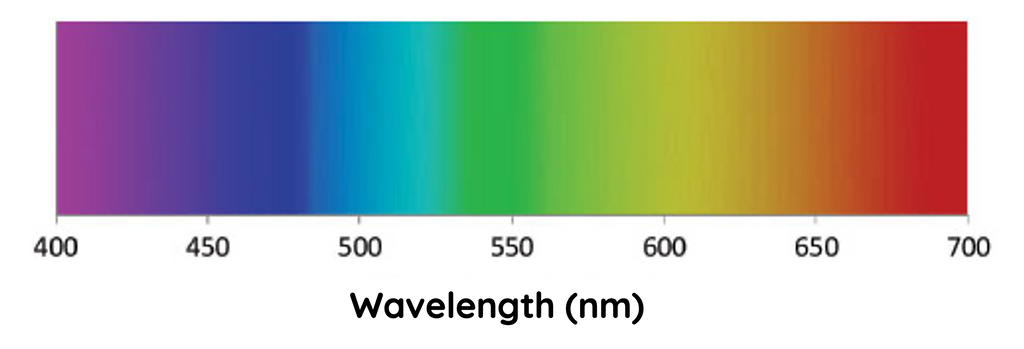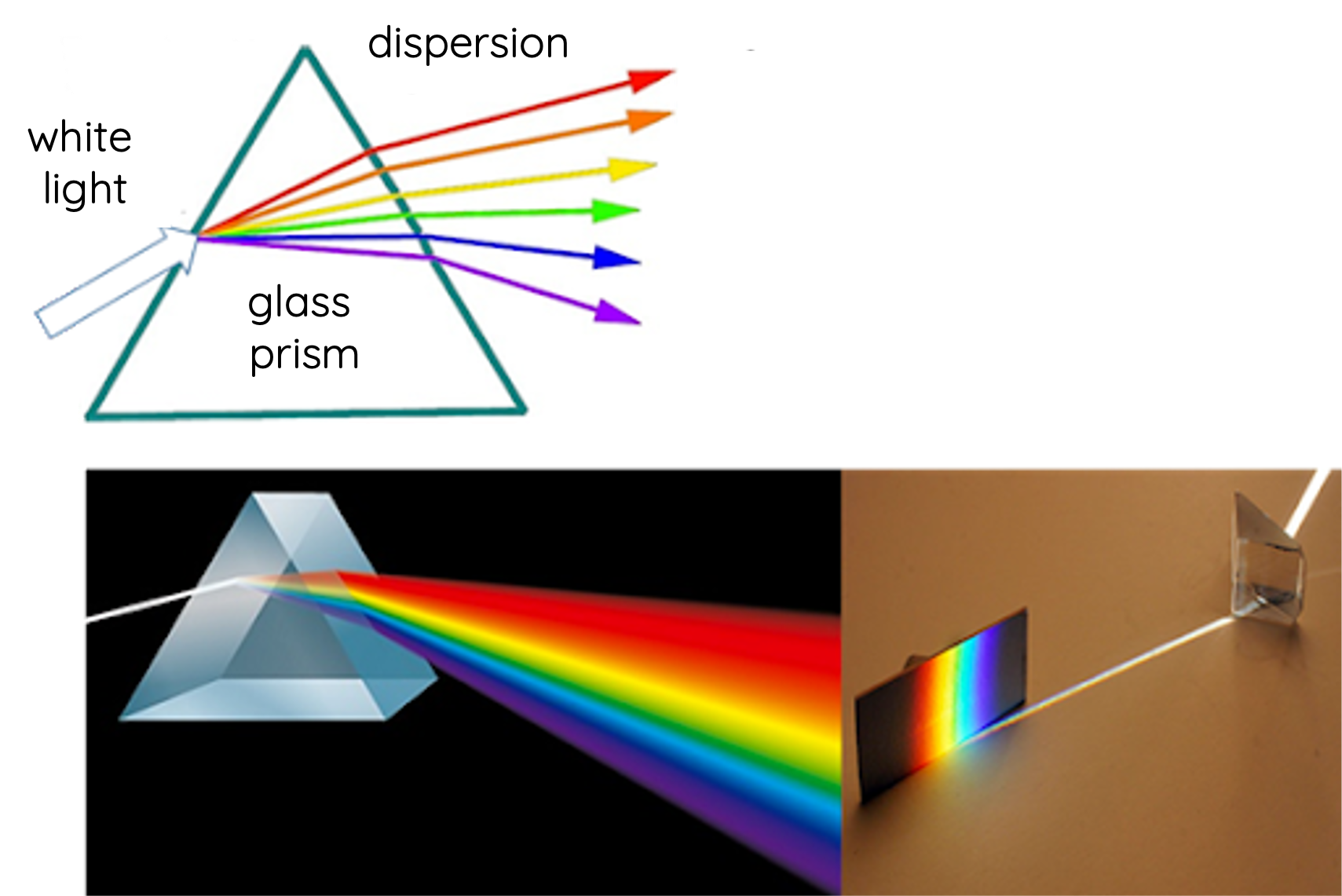# Dispersion of Light

This topic is part of the HSC Physics course under the section Ray Model of Light.

### HSC Physics Syllabus

• conduct a practical investigation to demonstrate and explain the phenomenon of the dispersion of light

### What is White Light?

White light refers to light that is a combination of all the visible colours of the spectrum. In a sense, it's a mixture of various wavelengths of light that human eyes can detect, ranging approximately from 400 nm (violet) to 700 nm (red).### What is Dispersion?

Dispersion refers to the separation of white light into its constituent colours (spectrum) due to the different frequencies (colours) of light in a medium, typically a transparent material like glass or water.

### Why Does Dispersion Occur?

The root cause of dispersion lies in refraction, the bending of light as it travels from one medium to another. However, not all wavelengths of light bend by the same amount. In most transparent materials, shorter wavelengths (blue and violet) refract more than longer wavelengths (red and yellow). This differential refraction is what causes light to spread out into its constituent colours.

### Snell's Law

Snell's Law relates the angle of incidence and the angle of refraction to the refractive index of a medium. It's given by:

$$\frac{n_2}{n_1} = \frac{\sin(\theta_i)}{\sin(\theta_r)}$$

Since the velocity of light depends on the refractive index of the medium:

$$v_x = \frac{c}{n_x}$$

The velocity of light changes with the medium it propagates in because its wavelength changes (frequency remains constant).

Thus, we can derive an expression for Snell's law in terms of velocity and wavelength of light in a medium:

$$\frac{n_2}{n_1} = \frac{\sin(\theta_i)}{\sin(\theta_r)} = \frac{v_1}{v_2} = \frac{\lambda_1}{\lambda_2}$$

### Refractive Index Varies with Wavelength

The refractive index of a medium isn't a constant value but varies with the wavelength of the incoming light. This variation is particularly noticeable in transparent materials like glass or water.

Typically, shorter wavelengths (e.g., violet and blue light) have a higher refractive index and thus travel more slowly in a medium compared to longer wavelengths (e.g., red and yellow light). As a result of the higher refractive index, shorter wavelengths bend more (smaller angle of refraction) when entering a new medium than longer wavelengths.

Table shows different refractive indices for various media and wavelength of light.### Why Does Refractive Index Depend on Wavelength of Light?

The reason for the wavelength dependency of the refractive index lies in the atomic and molecular structure of the medium. When light encounters a medium, the electric field of the light causes the electrons in the medium to oscillate. The extent to which these electrons can oscillate in response to the light depends on the light's frequency (which is inversely related to its wavelength).

When the frequency of light is closer to the natural oscillation frequencies of the electrons in the medium, the light interacts more strongly and slows down more, leading to a higher refractive index. This is why different wavelengths, having different frequencies and wavelengths, experience different refractive indices and thus refract to different extents.

### Practical Demonstration with a Prism:

A classic way to observe and study the dispersion of light is by using a triangular glass prism.Procedure:

1. Darken the room and let a thin beam of sunlight or white light from a torch pass through the prism.
2. Observe the light as it exits the other side of the prism.

Observations: You'll see a beautiful spectrum of colours, commonly remembered by the acronym ROYGBIV:

• Red
• Orange
• Yellow
• Green
• Blue
• Indigo
• Violet

The red light is refracted the least, while the violet light is refracted the most.

Formation of a rainbow is an example of dispersion of sunlight when it pass through rain droplets.

### Calculation Example

A beam of white light goes from air into flint glass at an incidence angle of of 43.2º . Refractive index of flint glass for red and violet light are 1.662 and 1.698 respectively.(a) What is the angle between the red (660 nm) and violet (410 nm) parts of the refracted light?

(b) What is the velocity of the red light in flint glass?

(c) What is the wavelength of the red light in flint glass?

Solution to part (a):

$$n_1 \sin(\theta_1) = n_2 \sin(\theta_2)$$

$$\theta_2 = \sin^{-1}\left(\frac{n_1 \sin(\theta_1)}{n_2}\right)$$

For red light:

$$\theta_{2_{\text{red}}} = \sin^{-1}\left(\frac{1 \times \sin(43.2^\circ)}{1.662}\right)$$

$$\theta_{2_{\text{red}}} = 24.3º$$

For violet light:

$$\theta_{2_{\text{violet}}} = \sin^{-1}\left(\frac{1 \times \sin(43.2^\circ)}{1.698}\right)$$

$$\theta_{2_{\text{violet}}} = 23.8º$$

The difference between the angle of refraction between the red and violet light is given by:

$$\Delta\theta = \theta_{2_{\text{red}}} - \theta_{2_{\text{violet}}} = 24.3 - 23.8 = 0.548º$$

Solution to part (b):

$$v_x = \frac{c}{n_x}$$

$$v_x = \frac{3 \times 10^8}{1.662}$$

$$v_x = 1.8 \times 10^8 \, \text{m/s}$$

Solution to part (c):

$$\frac{n_2}{n_1} = \frac{\lambda_1}{\lambda_2}$$

$$\frac{1.662}{1} = \frac{660}{\lambda_2}$$

$$\lambda_2 = 397 \, \text{nm}$$

Note that colour of light depends on the frequency of light (rather than wavelength). Although the wavelength shortens, the colour of the red light remains unchanged in the new medium (glass).